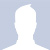-->

# SUMPRODUCT function in Excel

Welcome back

In today's lesson, we will soon learn about a very important Excel function with more than one example for clarification

# SUMPRODUCT function in Excel

At first we will get acquainted with a very important question

### What is the SUMPRODUCT function?

The SUMPRODUCT function returns the sum of the products of the matching ranges or arrays. The default operation is multiplication, but addition, subtraction, and division are also possible.

### How to use the SUMPRODUCT function?

In this example, we'll use SUMPRODUCT to return the total sales for certain items and volume

### syntax

To use the default operation (multiplication):

=SUMPRODUCT(array1, [array2], [array3], ...)

The SUMPRODUCT function syntax has the following arguments:

 argument description array1 wanted The first array argument you wish to multiply and then add its components. [array2], [array3],... choice Array arguments from 2 to 255 whose components you wish to multiply and then add.

### To perform other arithmetic operations

Use SUMPRODUCT as usual, but replace the commas separating the array arguments with the arithmetic operators you want (*, /, +, -). After all operations are performed, the results are collected as usual.

#### Notes

·         Array arguments must have the same dimensions. If you don't, SUMPRODUCT #VALUE! It is an error value. For example, =SUMPRODUCT(C2:C10,D2:D5) returned an error because the domains are not the same size.

·         SUMPRODUCT treats non-numeric array entries as if they were zeros.

·         For best performance, SUMPRODUCT should not be used with full column references. Think =SUMPRODUCT(A:A,B:B), here the function will multiply the 1048576 cells in column A by the 1048576 cells in column B before adding them.

#### Example 1

To create the formula using the sample list above, type =SUMPRODUCT(C2:C5,D2:D5) and press Enter. Each cell in column C is multiplied by its corresponding cell in the same row in column D, and the results are added up. The total amount of groceries is \$78.97.

To write a longer formula that gives you the same result, type =C2*D2+C3*D3+C4*D4+C5*D5 and press Enter . After pressing Enter, the result is the same: \$78.97. Cell C2 is multiplied by D2, and the result is added to the result of cell C3 times cell D3 and so on

#### Example 2

The following example uses SUMPRODUCT to return total net sales by sales agent, where we have both total sales and expenses by agent. In this case, we are using an Excel table that uses structured references instead of standard Excel ranges. Here you will see that the Sales, Expense, and Agent ranges are indicated by name.

The formula is: =SUMPRODUCT(((Table1[Sales])+(Table1[Expenses]))*(Table1[Agent]=B8)) , and it returns the sum of all sales and expenses for the factor listed in cell B8.

Related Articles

convert function

Index - Match Functionكاتب المقالة
كاتب ومحرر اخبار اعمل في موقع عالم الاوفيس .